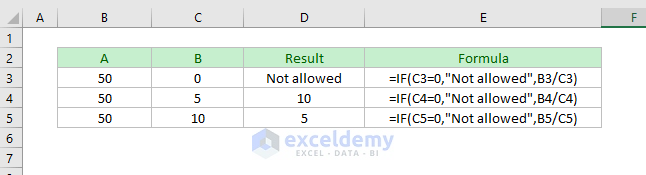Disclosure: This post may contain affiliate links, meaning when you click the links and make a purchase, we receive a commission.

# Division formula in Excel for multiple cells

Do you want to know how to use the division formula in Excel for multiple cells?

You are in the right place.

Let’s start…

## What is the division formula in Excel?

Actually, Excel does not have any DIVIDE function to perform the Division Operation.

Instead, you have to use the Forward Slash Operator (/) to divide two numbers or cells in Excel.

• 25/5 = 5
• A1/B1 = 5, where A1 and B1 hold numbers 50 and 10 respectively.
• A1/10 = 5, where A1 holds number 50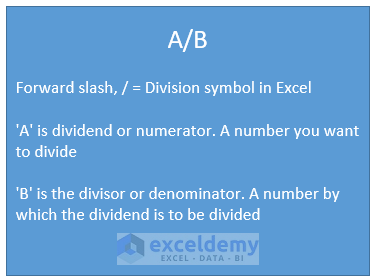## Division formula in Excel for Multiple Cells

You can use Excel’s division symbol to divide multiple cells in Excel.

For example,

A2/B2/C2 = 5, where A2 = 150, B2 = 3 and C2 = 10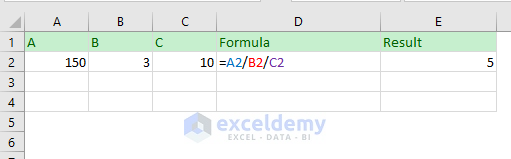How does this formula work?

In an Excel formula, division and multiplication have the same order of calculation but with the left to right associativity. Read this article to know more: Order & Precedence of Operations in Excel.

• So, at first, A2/B2 will be calculated = 150/3 = 50
• Then the result of A2/B2 (50) will be divided by C2 = 50/10 = 5

Now check out this image.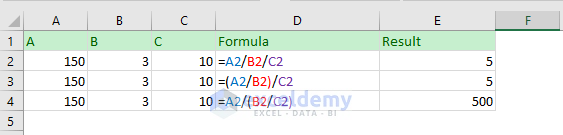You see that formula =A2/(B2/C2) has returned value 500. Why?

Because, according to calculation order, the expression inside a parenthesis will be evaluated first.

• So, (B2/C2) will be evaluated at first = 3/10 = 0.3333
• Next A2 will be divided by the result of B2/C2 (0.3333) = 150/0.3333 = 500

Hope this is clear to you.

## Divide two or more numbers using Forward Slash (/)

Dividing two or more numbers using Excel’s division symbol is the same as dividing two or more cells. See the image below.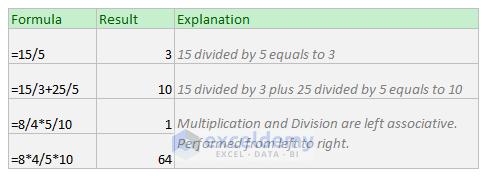Important to know:

Don’t forget to place an equal sign (=) before the formula; otherwise Excel might treat your entry as a date value. For example, if you input value 11/19, Excel will show your entry as 19-Nov. The cell value will be 11/19/2018 (the year I am writing this article).

Or, if you type 11/50, the cell might show value Nov-50. The cell will hold value 11/1/1950. This time as 50 cannot be a day value, Excel assumes that you’re typing the month and the year.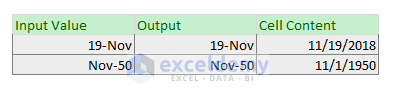## Divide the values of a column by a number (using formula)

### # Method 1: By a specific number

Suppose you want to divide the values of a column (multiple cells) using a specific number (say 10). Use these steps:

• In the cell B2, insert this formula: A2/10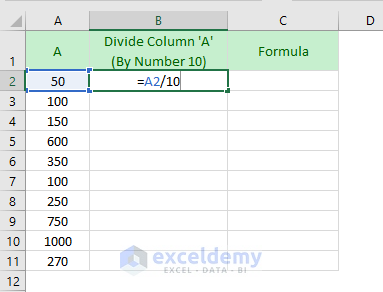• Now press Enter and copy this formula for other cells in the column.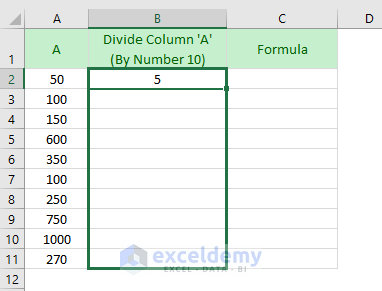• And here is the result. On the right side of the image, you are seeing the formulas.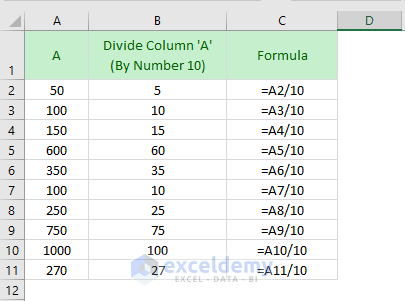### # Method 2: Dynamically

Now, what if you need to divide the above column with number 50?

Will you edit the formula by changing the number from 10 to 50, and then copy the formula for other cells in the column?

It is not a good idea.

We can write this formula newly. Check out the image below. We have written the formula using an absolute cell reference (\$B\$2).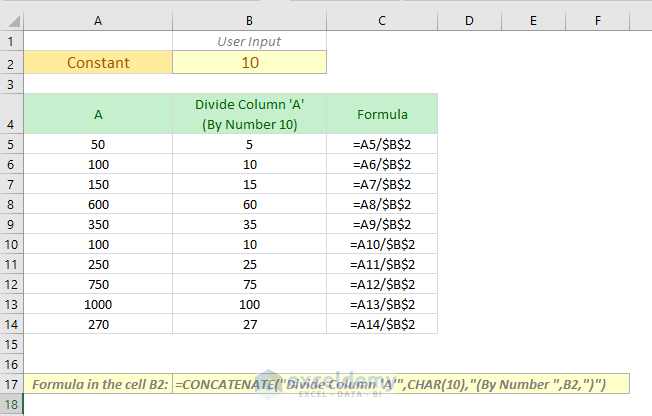By inserting different values in the cell B2, we can divide the column with different numbers.

Check out the following GIF image.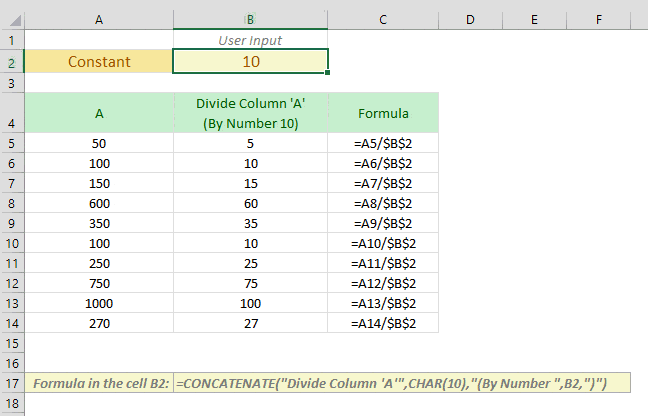You see that also the heading of the 2nd column is also changing (above image). To make the column heading dynamic, I have used this formula:

`=CONCATENATE("Divide Column 'A'",CHAR(10),"(By Number ",B2,")")`

CHAR(10) will insert a new line. And the CONCATENATE function joins the texts. Know more about the CONCATENATE function from this link.

## Divide the values of a column by a constant number (using Paste Special command)

If you want to divide the values of a column and want to keep the division values in the same column, then Paste Special is the way. Follow these steps:

• Place your divisor in a cell. In our case, we have placed the divisor in the cell B1 (with value 10). Select the cell and copy the value (CTRL + C).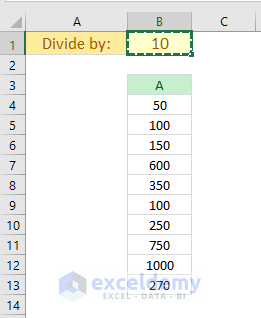• Now select the values of the column -> Right-click and then click on the Paste Special command from the menu.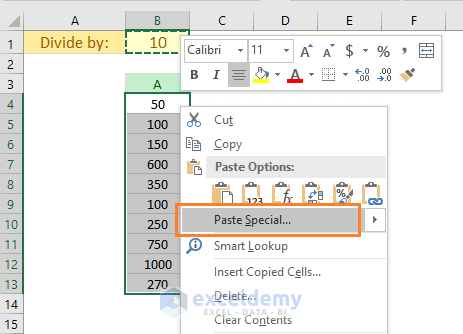• Paste Special dialog box appears. In the dialog box, select the Divide option (on the bottom-right corner of the dialog). And finally, click OK.

Keyboard shortcut to open the Paste Special dialog box: CTRL + ALT + V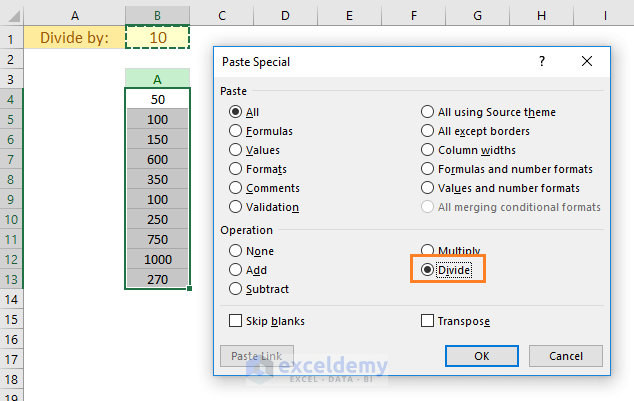• And this is what you get.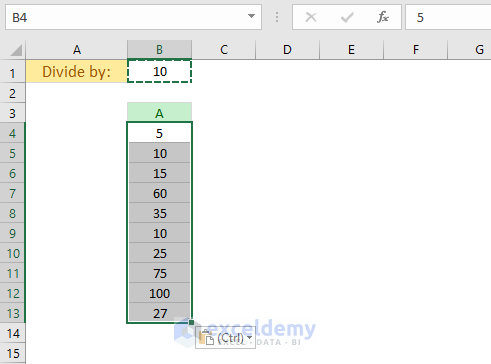You see that the results of the divisions have replaced the existing numbers.

## How do you divide by 1000 in Excel?

Suppose A1 = 10,000 and you want to divide A1 by 1000. You can use these ways:

• Using 1000 directly in the formula: A1/1000
• Place the number in a cell. I place the number in cell A2 (A2 = 1000). Now use this formula: A1/A2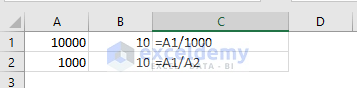## How do you add then divide in Excel?

This is a little bit tricky.

Suppose you want to add two numbers (say they are 50 and 60) and then want to divide the result with another number 11.

Here is the formula: (50 + 60)/11 = 110/11 = 10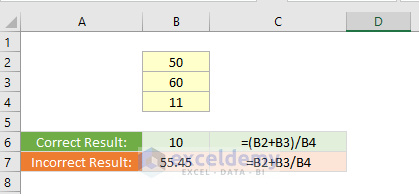If you write the formula in this way: 50 + 60/11, it will result = 50 + 5.45 = 55.45; you will not get the desired result. Because, according to the order of calculations, the division will perform first (with the left to right associativity) and then the addition.

So, you have to place the addition within a parenthesis. Parenthesis has a higher order of calculations than the division.

Want to know more about operator precedence and associativity in Excel? Read this article: What is the Order & Precedence of Operations in Excel?

## Calculate Percentage with Excel division formula

Suppose your company has total sales from 4 Areas: \$257,800

From Area 1, the sale is \$35,890

What is the contribution of Area 1 in the overall sale?

You have to calculate percentage (%) sale of Area 1 = 35890/257800 = 0.1392 = 0.1392 x 100% = 13.92%

Note: 100% = 100/100 = 1. We can multiply with any number.

In Excel, you will just change the cell format using Percent Style (%).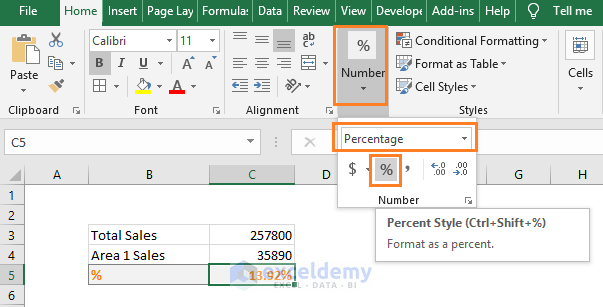## Handling #DIV/0! Error in Excel

In mathematics, you cannot divide a number by zero (0). It is not allowed.

So, when you divide a number by zero in Excel, it will show an error. It is #DIV/0! Error.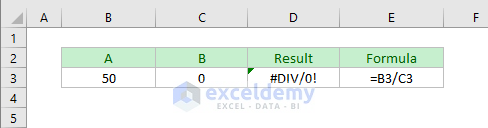We can handle #DIV/0! Error in Excel using two functions:

• IFERROR Function
• IF Function

### # Handling #DIV/0! Error using IFERROR function

At first, let’s use the IFERROR function to handle #DIV/0! Error.

Syntax of IFERROR Function: IFFERROR(value, value_if_error)

See how I have used the IFFERROR function to handle the #DIV/0! Error.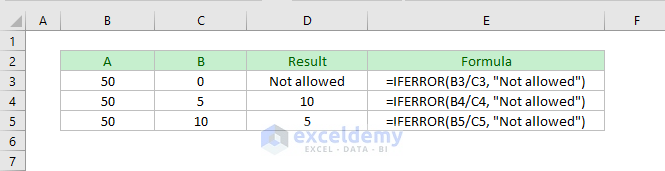### # Handling #DIV/0! Error using the IF function

Now, I will use the IF function to handle the #DIV/0! Error.

Syntax of IF function: IF(logical_test, [value_if_true], [value_if_false])

Here is the way to use IF function to handle #DIV/0! Error.